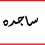# Newton's Three Laws of Motion

Newton's First Law of Motion:I. Every object in a state of uniform motion tends to remain in that state of motion unless an external force is applied to it.

Newton's Second Law of Motion:II. The relationship between an object's mass m, its acceleration a, and the applied force F is F = ma. Acceleration and force are vectors (as indicated by their symbols being displayed in slant bold font); in this law the direction of the force vector is the same as the direction of the acceleration vectorThis is the most powerful of Newton's three Laws, because it allows quantitative calculations of dynamics: how do velocities change when forces are applied. Notice the fundamental difference between Newton's 2nd Law and the dynamics of Aristotle: according to Newton, a force causes only a change in velocity (an acceleration); it does not maintain the velocity as Aristotle held.

This is sometimes summarized by saying that under Newton, F = ma, but under Aristotle F = mv, where v is the velocity. Thus, according to Aristotle there is only a velocity if there is a force, but according to Newton an object with a certain velocity maintains that velocity unless a force acts on it to cause an acceleration (that is, a change in the velocity). As we have noted earlier in conjunction with the discussion of Galileo, Aristotle's view seems to be more in accord with common sense, but that is because of a failure to appreciate the role played by frictional forces. Once account is taken of all forces acting in a given situation it is the dynamics of Galileo and Newton, not of Aristotle, that are found to be in accord with the observations.

Newton's Third Law of Motion:III. For every action there is an equal and opposite reaction.Note by Sagda Khalifa
7 years, 2 months ago

This discussion board is a place to discuss our Daily Challenges and the math and science related to those challenges. Explanations are more than just a solution — they should explain the steps and thinking strategies that you used to obtain the solution. Comments should further the discussion of math and science.

When posting on Brilliant:

• Use the emojis to react to an explanation, whether you're congratulating a job well done , or just really confused .
• Ask specific questions about the challenge or the steps in somebody's explanation. Well-posed questions can add a lot to the discussion, but posting "I don't understand!" doesn't help anyone.
• Try to contribute something new to the discussion, whether it is an extension, generalization or other idea related to the challenge.

MarkdownAppears as
*italics* or _italics_ italics
**bold** or __bold__ bold
- bulleted- list
• bulleted
• list
1. numbered2. list
1. numbered
2. list
Note: you must add a full line of space before and after lists for them to show up correctly
paragraph 1paragraph 2

paragraph 1

paragraph 2

[example link](https://brilliant.org)example link
> This is a quote
This is a quote
    # I indented these lines
# 4 spaces, and now they show
# up as a code block.

print "hello world"
# I indented these lines
# 4 spaces, and now they show
# up as a code block.

print "hello world"
MathAppears as
Remember to wrap math in $$ ... $$ or $ ... $ to ensure proper formatting.
2 \times 3 $2 \times 3$
2^{34} $2^{34}$
a_{i-1} $a_{i-1}$
\frac{2}{3} $\frac{2}{3}$
\sqrt{2} $\sqrt{2}$
\sum_{i=1}^3 $\sum_{i=1}^3$
\sin \theta $\sin \theta$
\boxed{123} $\boxed{123}$

Sort by:

How we should do Newton laws of motion and friction

- 6 years, 5 months ago

But they do not work inside a lift.

- 7 years, 1 month ago